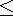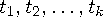Functions and CALL Routines

# DACCTAB Function

Returns the accumulated depreciation from specified tables.
 Category: Financial

## Syntax

 DACCTAB(p,v,t1, . . . ,tn)

### Arguments

p

is numeric, the period for which the calculation is to be done. For noninteger p arguments, the depreciation is prorated between the two consecutive time periods that precede and follow the fractional period.

v

is numeric, the depreciable initial value of the asset.

t1,t2, . . . ,tn

are numeric, the fractions of depreciation for each time period with t1+t2+...tn1.

The DACCTAB function returns the accumulated depreciation by using user-specified tables. The formula for this function isFor a given p, only the argumentsneed to be specified with k=ceil(p) .

An asset has a depreciable initial value of \$1000 and a five-year lifetime. Using a table of the annual depreciation rates of .15, .22, .21, .21, and .21 during the first, second, third, fourth, and fifth years, respectively, the accumulated depreciation throughout the third year can be expressed as

`   y3=dacctab(3,1000,.15,.22,.21,.21,.21);`

The value that is returned is 580.00. The fourth rate, .21, and the fifth rate, .21, can be omitted because they are not needed in the calculation.Previous Page | Next Page | Top of Page